# SECTION 4 - GEOMETRIC DISTORTION

## 4.1 OVERVIEW

Raw IUE images suffer from geometric distortion introduced by the SEC vidicon cameras. The electrostatically-focused image section of the camera produces a pincushion distortion, while the magnetically-focused readout section produces an S-distortion. An important part of the processing for each image is compensation for these geometric distortions. Although the reduction of spectral images under the current software no longer includes the explicit generation of a geometrically corrected image (except as a step in the creation of dispersion constants from wavelength calibration images and intensity transfer functions (ITFs) from flat-field images - see Figure 1-1, and Sections 5 and 6 ), implicit compensation for the geometric distortion of the raw image is still required. To do this, positions of fiducial marks (reseaux) are used, as they have been in the past, to map the distortion across the image (see, for example, Thompson et al., 1980). In Section 4.2 details regarding the measurement and modeling of reseau motion are discussed, and in Sections 4.3 and 4.4 the methods used to parameterize the geometric distortion and compensate for it in production processing are presented.

## 4.2 RESEAU MARKS (FIDUCIAL GRID)

The faceplate of each camera is etched with a square-grid pattern of 169 reseau marks arranged in 13 rows and 13 columns. The reseaux appear on images as occulted areas approximately 2-3 pixels wide spaced approximately 55 pixels apart (56-pixel separation for SWP, 55 pixels for LWP and LWR). According to the design specifications of the scientific instrument (GSFC System Design Report for the IUE, 1976), the placement of the reseau marks in a true square grid is accurate to within ± 0.005 mm, which corresponds to ± 0.14 pixels. Once the positions of the reseau marks are measured on raw images (approximately 129 of the 169 marks fall within the camera target area), the departure of the observed positions from their true locations is used to characterize the geometric distortion of the camera system.

### 4.2.1 MEASUREMENT OF RESEAU POSITIONS

Because reseau marks are in general poorly visible on all data images except those with a substantial background level, information on reseau positions used to model the geometric distortion for production processing has standardly been obtained from measurements on flood-lamp exposures. Perry and Turnrose (1977) provide a detailed description of the two-dimensional cross-correlation search methods used to locate the position of individual reseau marks on such exposures. Improvements to software and procedures have been subsequently made which affect various aspects of the reseau measurement process and have been documented in Turnrose and Harvel (1982) and Turnrose, Thompson and Gass (1984). These improvements made it possible to measure reseaux on low dispersion (and to a certain extent high dispersion) wavelength calibration (WAVECAL) images and basically include improved starting search positions, smaller search areas, and the ability to "fill in" (using linear interpolation) reseau positions which are not measurable due to superimposed Pt-Ne emission lines. As a result, flat-field images are no longer used for monitoring reseau motion and WAVECAL images are geometrically corrected using reseau positions measured on the low dispersion WAVECAL images.

The above improvements also made it possible to expand the analysis of reseau motion to include reseaux measured on low dispersion spectral images when the background level is above approximately 40 DN. These new studies may ultimately change the methods for compensating for geometric distortion used in production processing (see Section 4.2.2.3.2).

### 4.2.2 PARAMETERIZATION OF RESEAU MOTION

Various studies of reseau motion as a function of time, temperature and image intensity have been made over the last several years. One of the first studies was that by Oliver et al. (1979), which showed that shifts in reseau positions on flat-field images could be correlated with both image intensity and camera temperature. Later studies were primarily intended to determine methods for compensating for this reseau motion in the production processing of IUE images. For this reason studies were made of 60 percent ultraviolet floodlamp (UVFLOOD) images (Thompson, Turnrose and Bohlin, 1982a), tungsten floodlamp (TFLOOD) images and ITF UVFLOOD images (Thompson 1983a, 1984b) and most recently, low dispersion spectral images (Thompson 1984c).

Although production processing currently uses only the results described in Thompson, Turnrose and Bohlin (1982a) (see Section 4.3.1) the following sections will describe the latest conclusions regarding the modeling of reseau motion as a function of time, temperature, and image intensity.

### 4.2.2.1 Correlation with Time

Several problems exist in determining whether reseau motion is correlated with time (i.e., date of observation), the most important of which is that there does not exist a suitable set of images which (a) were obtained under similar conditions of camera temperature and exposure level and (b) cover the entire time period from launch to present. The first study of time dependence was by Thompson, Turnrose and Bohlin (1982a) in which 60 percent UVFLOOD images acquired over the first two years of IUE operation were examined for both temperature and time dependence. Since the 60 percent UVFLOODs are all exposed to the same intensity level, shifts due to beam-pulling effects (see Section 4.2.2.3) were minimized. A result of this study was that no evidence for secular variation was found.

The question of time dependence was studied again by Thompson (1983a) using TFLOOD images (~50 images each for LWR and SWP, ~25 images for LWP) and an extended set of 60 percent UVFLOOD images. In this study a correlation with time was discovered for the LWR UVFLOOD images. It was decided, however, that the apparent correlation was probably due to a beam-pulling effect resulting from a variation in the LWR UVFLOOD lamp itself, since the correlation was not seen with the TFLOOD images.

The TFLOOD images, which cover the period from 1980 to present, represent the largest consistent set of images available for monitoring reseau motion as a function of time. No significant correlation with time has been seen for these images. Any variation in reseau positions with time must be significantly less important than the correlations found with temperature and image intensity described below.

### 4.2.2.2 Correlation with Temperature

As discussed in detail in Thompson, Turnrose and Bohlin (1982a), the measured positions of reseau marks on 60 percent UVFLOOD images change from image to image due to a thermal sensitivity of the camera readout electronics (Oliver et al., 1979). These shifts, which are non-uniform across the image, range up to a maximum of about 1.5 pixels at the edge of the SWP tube for a change in THDA of 9C, have been correlated with camera head amplifier temperature (THDA) for the LWR and SWP cameras. Linear regressions for the reseau positions versus THDA have been established separately in the line and sample directions for both the SWP and the LWR cameras. When all reseaux within the target ring are considered, the average 1 sigma root-mean-square (rms) scatter in position can be reduced from 0.29 to 0.19 pixels in SWP and from 0.24 to 0.21 pixels in LWR by applying temperature-dependent corrections to the UVFLOOD reseau positions. Further information in tabular and graphical form may be found in Section 4.3.

The temperature correlations found with the 60 percent UVFLOOD images were verified in a later analysis which included studies of both TFLOOD and UVFLOOD reseau motion (Thompson 1983a). This study showed that an increase in THDA resulted in a contraction of the overall reseau grid for both the LWR and SWP cameras with the largest motion occurring in the SWP camera. A subsequent study (Thompson 1984b) showed that LWP TFLOOD reseaux exhibited a slight temperature dependence similar to that of the LWR reseaux.

### 4.2.2.3.1 Floodlamp Images

The study of Oliver et al. (1979) showed that reseau position shifts as large as 1.5 pixels can be induced by "beam pulling effects". As explained in that reference, the read beam is influenced by the charge distribution on the target, so that for relatively highly exposed areas the apparent position of a given pixel in the resulting image is shifted slightly in the general direction of the top of the image. Their study concluded that (a) beam pulling effects are largely confined to the immediate vicinity (i.e., within a few pixels) of a feature and (b) for weak features extending over relatively large areas, a much smaller long-range effect can be detected over distances on the order of 100 pixels.

The localized nature of the strongest beam-pulling effects has made compensation difficult, if not impossible. Partly for this reason, most of the reseau motion studies which followed that of Oliver et al. intentionally used sets of images in which neither short-range nor long-range beam pulling effects contributed to the relative reseau motion. Therefore, the studies based on TFLOOD and 60 percent UVFLOOD reseau positions, although useful for evaluating temperature and time correlations, do not provide any information on beam-pulling effects. Because the geometric correction in production processing is based on results from the study of 60 percent UVFLOOD images (see Section 4.3), some systematic errors are probably being introduced. Section 6.5 contains a discussion of the magnitude of such systematic effects as they pertain to wavelength assignments. An attempt to parameterize the reseau motion due to beam-pulling effects was recently made as a result of a study of ways to improve the geometric correction of ITF images. Correlations of reseau motion as a function of mean DN level and temperature were determined for a series of ITF UVFLOOD images taken at different exposure levels. After application of the deduced DN and temperature correlations the resulting residual scatter in reseau positions among three separate series of ITF images was always less than 0.08 pixels, which is less than that found from any other study (see Thompson 1983a).

### 4.2.2.3.2 Spectral Images

As a result of the above findings (and because of the limited number of available ITF UVFLOOD images), an on-going study of reseau motion in low dispersion spectral images was initiated. As reported by Thompson (1984c), results show that for each camera, a correction based on a mean DN level is more significant in reducing the residual scatter than a correction for temperature. The continuing work in this study will most likely result in an improvement to current production processing procedures in the near future.

## 4.3 RESEAU DISPLACEMENT FILES

As actually utilized in production processing, the reseau positions are expressed in terms of displacements which are the differences between the reseau positions as found on raw images and the actual 13 x 13 grid of reseau marks located between the UV converter and the SEC vidicon camera. Displacements for reseaux lying outside of the camera target area are calculated by linear extrapolation of the measured displacements. As explained in Section 4.4 it is these displacement files (after the application of any parameterized corrections as described below) that are used by the IUESIPS application programs to compensate for the geometric distortion in IUE images.

### 4.3.1 IMPLEMENTATION IN PRODUCTION PROCESSING

Prior to mid-July 1980 the displacement files used in production processing were based on smoothed reseau positions obtained from TFLOOD exposures acquired approximately every two weeks. Chebyshev polynomials were used to smooth the rows and columns of the reseau grid prior to the generation of the displacement files. After the above date, the displacement files were created from averages of reseau positions measured on 60 percent UVFLOOD exposures, without applying the polynomial smoothing mentioned above. The advantages to this method are described in Thompson et al. (1980). A correction for thermal shifts for the SWP camera was finally implemented in March 1981 for low dispersion images and in May 1981 for high dispersion images as explained in Thompson, Turnrose and Bohlin (1982a). The following sections describe in more detail the displacement files currently used for each camera in production processing.

### 4.3.1.1 LWR

Because the overall improvement for LWR reseau positions after temperature correction was so marginal (as explained in Section 4.2.2.2), and because there are no large local deviations, the THDA correction has not been applied in LWR production processing. The lack of improvement is shown graphically in Figure 4-1 which shows, for each reseau, the 1 sigma scatter before and after correction in the directions along and perpendicular to the high dispersion orders.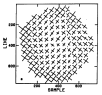Figure 4-1a. a. Scatter in the Two Orthogonal Directions (Along and Perpendicular to High Dispersion Orders) for the Reseau Locations as Observed on 15 LWR Flat-Field-Images. The Length of the Bars Represents >1s. (Same as Figure 5a of Thompson, Turnrose, and Bohlin 1982a).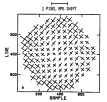Figure 4-1b. b. As Above, but After Applying Temperature Corrections. (Same as Figure 5b of Thompson, Turnrose, and Bohlin 1982a).

The LWR displacement file used in production processing was generated using the mean reseau positions of 15 60 percent UVFLOOD images (Thompson, Turnrose and Bohlin 1982a). These mean displacements (i.e., mean found minus actual positions) are shown in Table 4-1. The actual sample positions are the first row of integers at the top of the tables, while the actual line numbers are the first column. The displacements at each actual reseau position in Table 4-1 are listed in sample (upper entry) and line (lower entry) pairs. The mean displacements are illustrated graphically in Figure 4-2. In this display, the actual positions of the 169 reseaux are marked by diamonds; the displacements, magnified for visual clarity by a factor of two, are indicated by the vectors. The amount of distortion is therefore exaggerated for display, although the directions and relative proportions of the displacements are correct.

### 4.3.1.2 SWP

Because the overall improvement for SWP reseau positions after temperature correction is significant and because even larger local improvements are realized, the THDA correction is applied in SWP production processing, based on the THDA at the time the image is read, as extracted from the camera snapshot portion of the image science header. If the THDA at the time of read cannot be extracted from an image header, the processing defaults to the mean reseau displacements (given in Table 4-2 and shown graphically in Figure 4-3 as for LWR) unless a specific THDA value is manually specified by the operator when the image is processed.

The temperature-corrected SWP reseau displacements are equal to a value R(s) samples and R(l) in lines determined from

(4-1)
R(s) = R1(s) + R2(s) × THDA
R(l) = R1(l) + R2(l) × THDA

where the R1 and R2 values are given in Tables
4-3 and 4-4 in the position corresponding to the same line or sample displacement in Table 4-2. The improvement due to temperature correction is seen in Figure 4-4 which displays the 1s scatter before and after correction in the same form as shown for LWR in Figure 4-1.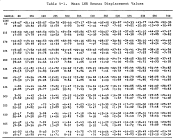Table 4-1: Mean LWR Reseau Displacement Values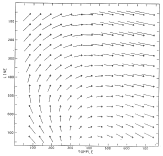Figure 4-2: Displacements of Mean Reseaux from Correct Grid LWR (Magnified by 2).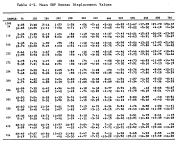Table 4-2: Mean SWP Reseau Displacement Values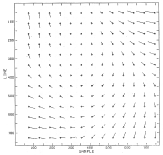Figure 4-3: Displacements of Mean Reseaux from Correct Grid SWP (Magnified by 2).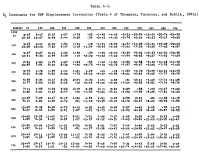Table 4-3: R1 Constants for SWP Displacement Correction (Table 4 of Thompson, Turnrose, and Bohlin (1982a)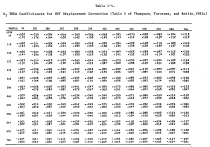Table 4-4: R2 THDA Coefficiencts for SWP Displacement Correction (Table 5 of Thompson, Turnrose, and Bohlin 1982a)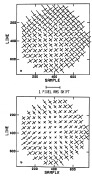Figure 4-4: a. Scatter in the Two Orthogonal Directions (Along and Perpendicular to High Dispersion Orders) for the Reseau Locations as Observed on 18 SWP Flat-Field Images. The length of the Bars Represents > 1 sigma. (Same as Figure 4a of Thompson, Turnrose, and Bohlin 1982a).

b. As above, but After Applying Temperature Corrections. (Same as Figure 4b of Thompson, Turnrose, and Bohlin 1982a).

### 4.3.1.3 LWP

The current LWP displacement file was generated from an average of three 60 percent UVFLOOD images obtained in 1980. As for the other cameras, these mean displacements are shown in Table 4-5 and graphically in Figure 4-5. The limited number of available LWP UVFLOOD images prevented more images from being included in the analysis and prevented any correlations with time, THDA, or DN from being determined.

### 4.3.1.4 SWR

The SWR is not an operational camera at this time.

### 4.3.2 DOCUMENTATION IN IMAGE LABELS

Information identifying the parameters pertinent to the geometric compensation applied to any particular image is documented by entries made in the processing history portion of the image label, in a format described in Section 9. This information includes an identifier for the reseau set used, the THDA value used for temperature correction, if appropriate, and other relevant data.

## 4.4 DISTORTION COMPENSATION IN PRODUCTION PROCESSING

Based upon the reseau displacements appropriate to each image and computed as described in the preceding sections, the geometric distortion in that image is compensated for during processing by a mapping function which calculates interpolated displacement values at any point within the image. This mapping function establishes the correspondence between points in the raw (distorted) image and points in a geometrically correct (undistorted) image. If we designate the function which transforms sample and line coordinates (s,l) in the raw image to sample and line coordinates (x,y) in the geometrically correct image as

(4-2)
(x,y) = G(s,l)

(following Lindler, 1982a) then the inverse function G-1 transforms geometrically correct coordinates to raw coordinates:

(s,l) = G-1(x,y)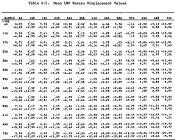Table 4-5: Mean LWP Reseau Displacement Values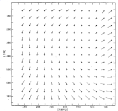Figure 4-5: Displacements of Mean Reseaux from Correct Grid LWP (Magnified by 2).

Because the function G-1 is derivable directly from the reseau displacement file by interpolation, it is used throughout the new software system to actually perform the geometric mapping. In certain situations, however, the function G is a conceptually convenient tool to use in explaining geometric relationships and will be used in several discussions in succeeding sections.

### 4.4.1 GEOM-TO-RAW MAPPING FUNCTION G-1

Following Lindler (1982a), let dij represent the displacement vector of the reseau marks in row i, column j of the reseau grid. To find the position (s,l) in raw space corresponding to the arbitrary position (x,y) in geometrically correct space, one needs to calculate the displacement vector d = (dx,dy) for the point (x,y). This is done via a bilinear interpolation of the reseau displacements, using the following steps.

1. Determine the four reseau positions between which the point (x,y) lies. That is, if xj is the sample coordinate for reseau column j and yi is the line coordinate for reseau row i in geometrically correct space, then determine i and j such that

(4-4)
xj < x < xj+1
yi < y < yi+1

2. Compute the fractional distances u and v:
u = (x - xj) ÷ (xj+1 - xj)
v= (y - yi) ÷ (yi+1 - yi)
3. Compute the displacement d by interpolation:
d = (dx,dy) = (1-u)(1-v)di,j + (1-u)vdi+1,j + u(1-v)di,j+1 + uvdi+1,j+1

Having calculated the appropriate displacement vector d, one can then compute the sample and line coordinates in raw space:

(s,l) = (x+dx,y+dy) = G-1(x,y)

Under the old IUE software, the function G-1 was used to perform the mapping needed for doing the explicit geometric correction of images. Although this explicit correction is now done only for wavelength calibration and certain flat-field images as mentioned in Section 4.1, the same function G-1 is still used in the new software to refer positions associated with geometrically correct space back to the corresponding raw-image positions.

### 4.4.2 RAW-TO-GEOM MAPPING FUNCTION G

The inverse of the function G-1 is the function (G-1)-1 = G. Although, as explained earlier, the function G is not used algorithmically in the actual software, it is conceptually useful in describing the relationship between raw-image space and the geometrically corrected intensity transfer function in the context of the photometric correction process (see Section 5).

### 4.4.3 ACCURACY OF DISTORTION COMPENSATION

In general, the accuracy of the compensation for geometric distortion is not directly measured. However, errors in the distortion compensation can be seen by their effect on the wavelength assignment accuracy (Section 6.5), the photometric correction (Section 5), the registration procedure described in Section 6.3.2, and the accuracy of the gross and background flux extraction in high dispersion (Section 7.1).

The errors involved can derive from both (a) errors in the found reseau positions used and (b) small-scale distortions which are not corrected by simple linear interpolation between the reseau positions. The latter error is seen as "wiggles" in the spectral orders and has been described by several authors; see Panek (1982b), de Boer and Meade (1981), Thompson (1982), and Cassatella, Barbero and Benvenuti (1983).